# z Parameters of Two Port Network

## z Parameters of Two Port Network:

z Parameters of Two Port Network are also called impedance parameters. These are obtained by expressing voltages at two ports in terms of currents at two ports. Thus, currents I1 and I2 are independent variables; while V1 and V2 are dependent variables. Thus, we have,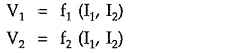In equation form, above relations can be written as,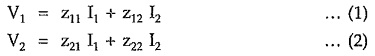In matrix form, above equations can be written as,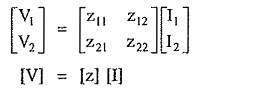The individual z-parameters are defined as follows.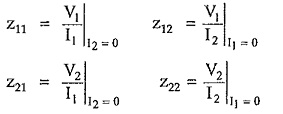From above equations it is clear that Z11 and Z22 gives the relationship between voltages and currents at port 1 – 1′ and port 2 – 2′ respectively. Such parameters are the driving point impedances. While Z12 and Z21 gives the relationship between voltage and currents at different ports. Such parameters are the transfer impedances.

Moreover, these parameters are defined only when the current in one of the ports is zero. This corresponds to the conditions that the one of the ports is open circuited. Hence z-parameters are named as open circuit impedance parameters.

Hence we can write,

• Z11 = circuit driving point input impedance
• Z22 = Open circuit output impedance
• Z12 = Open circuit forward transfer impedance
• Z21 = Open circuit reverse transfer impedance.

The equivalent circuit of a two port network interms of open circuit impedance parameters satisfying equations (1) and (2) is as shown in the Fig. 6.3.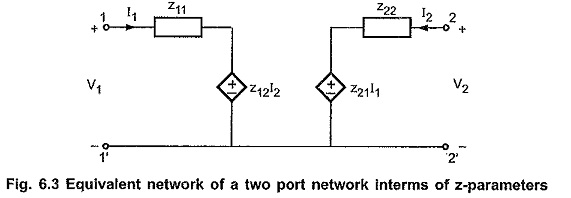Updated: November 4, 2019 — 11:04 pm